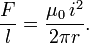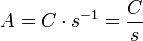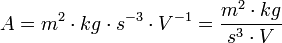# Ampere (unit)

The ampere, symbol A, is the SI unit of electric current. It is defined by application of Ampere's equation:$\frac{F}{l} = \frac{\mu_0\,i^2}{2\pi r}.$

One ampere is that constant current i which, if maintained in two straight parallel conductors of infinite length, of negligible circular cross-section, and placed r = 1 metre apart in vacuum, would produce between these conductors a force F equal to 2 x 10-7 newton per meter of length l. This sets the value of the magnetic constant μ0 to 4π x 10−7 N/A2.

The ampere is named for André-Marie Ampère, an early investigator of electricity, magnetism, and chemistry.

The ampere has undergone a number of redefinitions; the current standard was adopted in 1948. One definition adopted legally before the current SI definition was "that unvarying current that would deposit 0.001 118 000 grams of silver per second from a solution of silver nitrate in water". This earlier definition is approximately 0.99985 A (SI).

## Related units

The SI uses the ampere as its basic unit of electrical measure; all other units are derived from the ampere.

• The coulomb is the unit of electrical charge, and is equal to the amount of charge passing a point in one second in a circuit with one ampere of current.$A=C\cdot s^{-1}=\frac{C}{s}$$A = m^2 \cdot kg \cdot s^{-3} \cdot V^{-1} = \frac{ m^2 \cdot kg}{s^{3} \cdot V}$.
• The ohm is the unit of electrical resistance, and is the resistance which will allow a current of one ampere across a potential drop of one volt.$A=V\cdot \Omega^{-1}=\frac{V}{\Omega} = \sqrt{m^2 \cdot kg \cdot s^{-3}\, \Omega^{-1}}$
• The farad is the unit of electrical capacitance, and is the capacitance of a capacitor whose potential between the plates increases by one volt when charged with one coulomb of charge.